Courses

# Digital Electronics - MCQ Test 1

## 15 Questions MCQ Test Mock Tests Electronics & Communication Engineering GATE 2020 | Digital Electronics - MCQ Test 1

Description
This mock test of Digital Electronics - MCQ Test 1 for GATE helps you for every GATE entrance exam. This contains 15 Multiple Choice Questions for GATE Digital Electronics - MCQ Test 1 (mcq) to study with solutions a complete question bank. The solved questions answers in this Digital Electronics - MCQ Test 1 quiz give you a good mix of easy questions and tough questions. GATE students definitely take this Digital Electronics - MCQ Test 1 exercise for a better result in the exam. You can find other Digital Electronics - MCQ Test 1 extra questions, long questions & short questions for GATE on EduRev as well by searching above.
QUESTION: 1

### What are the essential prime implicants of following Boolean function x'z + y`z + xz'

Solution:

flx, y, z)= xlz + ylz + xzl These pairs are essential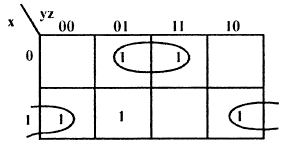QUESTION: 2

### Consider a multiplexer with X &Y as data inputs and z as a control pin. If Z = 0, selects input X and Z = I , selects input Y what are the connections required to realize the 2 - variable Boolean functions f T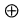R

Solution: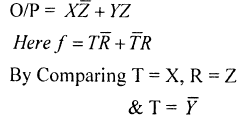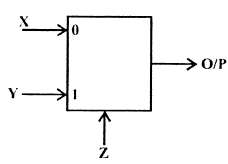QUESTION: 3

### A combinational circuit, using 8 x 1 MUX is shown in figure. The minimized expression for output Z is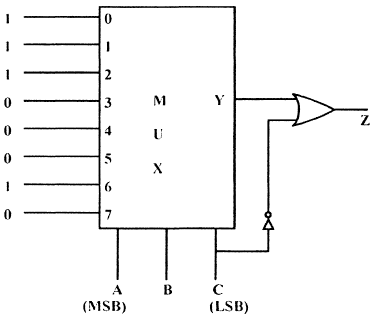Solution: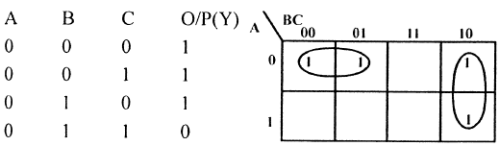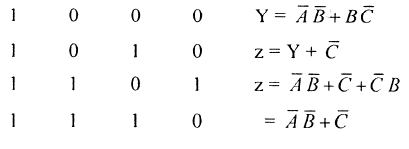QUESTION: 4

The Minimal Product of sums function described by k-Map, shown in figure is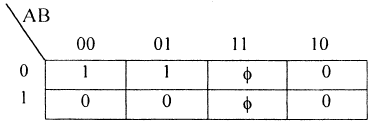Solution: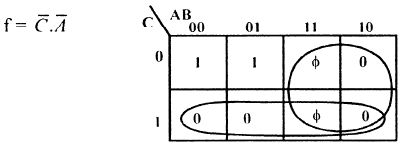QUESTION: 5

The output of an AND gate with three inputs, A, B, and C, is HIGH when ________.

Solution:
QUESTION: 6

The digital circuit using two inverters shown in figure will acts as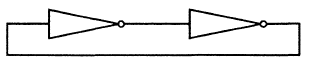Solution:

When 2 inverters are connected then it will become bi-stable.

QUESTION: 7

For the TTL. circuit shown in figure i/Ps are floating, if floating i/Ps are A, B, C and D then what is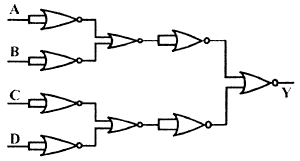value of output Y of circuit.

Solution:

TTL floating input is I .

*Answer can only contain numeric values
QUESTION: 8

If 2's complement representation of a 16-bit number (one-sign bit and 15 Magnitude bits) is FFFFthen its magnitude in decimal representation is ____

Solution: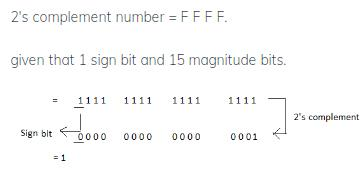QUESTION: 9

The initial state of MOD — 16, down counter is 0110. What state will be it be after 37 clock pulses??

Solution: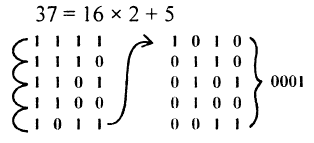QUESTION: 10

In the given figure shows a negative logic AND gate. If positive logic is used this gate is equivalent to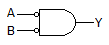Solution:

Y = A B = A + B .

QUESTION: 11

An 8085 Microprocessor based system uses a 4 k x 8 bit RAM whose starting address is AAOOH. The address of last byte in this RAM is

Solution:

4K x 8 = 212 x 8 bit it has 12 bits So address of last - byte will be AA 00 + 0 FFF = B9FF

QUESTION: 12

What is F's complement of (2BFD)16

Solution:

F'S complement of 2BFD is FFF F - 2BFD = D402

QUESTION: 13

A. ROM is interfaced to an 8085 CPU as indicated in figure, shown, what is address range occupied by ROM is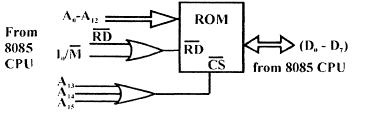Solution:

A15 A 14 A 13 will be 000 So Range will be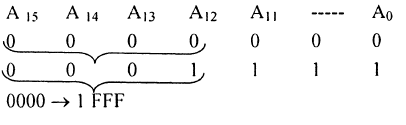QUESTION: 14

112 4-bit 2's complement no's 1011 and 0110 are added, then result expressed in 2's complementnotation is

Solution:
QUESTION: 15

Which one of the following statement is correct?

Solution: